# Minimum + maximum - math problems

#### Number of problems found: 25

• Camp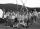In a class are 26 children. During the holidays 16 children were in the camps and 14 children on holiday with their parents. Determine the minimum and maximum number of children that may have been in the camp and on holiday with their parents at the same
• DigitsWrite the smallest and largest 2-digit number.
• Cone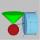Into rotating cone with dimensions r = 8 cm and h = 8 cm incribe cylinder with maximum volume so that the cylinder axis is perpendicular to the axis of the cone. Determine the dimensions of the cylinder.
• Statue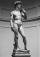On the pedestal high 4 m is statue 2.7 m high. At what distance from the statue must observer stand to see it in maximum viewing angle? Distance from the eye of the observer from the ground is 1.7 m.
• ClassmatesRoman is ranked 12th highest and eleventh lowest pupil. How many classmates does Roman have?
• Trousers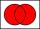In the class was 12 students. Nine students wearing trousers and turtleneck eight. How many students worn trousers with a turtleneck?
• Glasses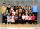Imagine a set of students in your class (number of students: 19), who wears glasses. How much minimum and maximum element may contain this set.4 m long ladder touches the cube 1mx1m at the wall. How high reach on the wall?
• Cylindrical containerAn open-topped cylindrical container has a volume of V = 3140 cm3. Find the cylinder dimensions (radius of base r, height v) so that the least material is needed to form the container.
• Skoda carsThere were 16 passenger cars in the parking. It was the 10 blue and 10 Skoda cars. How many are blue Skoda cars in the parking?
• ShapePlane shape has a maximum area 677 mm2. Calculate its perimeter if perimeter is the smallest possible.
• Ten boys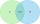Ten boys chose to go to the supermarket. Six boys bought gum and nine boys bought a lollipop. How many boys bought gum and a lollipop?
• Derivative problemThe sum of two numbers is 12. Find these numbers if: a) The sum of their third powers is minimal. b) The product of one with the cube of the other is maximal. c) Both are positive and the product of one with the other power of the other is maximal.
• Curve and lineThe equation of a curve C is y=2x² -8x+9 and the equation of a line L is x+ y=3 (1) Find the x co-ordinates of the points of intersection of L and C. (2) Show that one of these points is also the stationary point of C?
• Paper boxHard rectangular paper has dimensions of 60 cm and 28 cm. The corners are cut off equal squares and the residue was bent to form an open box. How long must be side of the squares to be the largest volume of the box?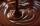In the box were total of 200 cookies. These products have sugar and chocolate topping. Chocolate topping is used on 157 cookies. Sugar topping is used on 100 cakes. How many of these cookies has two frosting?
• Secret treasureScouts have a tent in the shape of a regular quadrilateral pyramid with a side of the base 4 m and a height of 3 m. Determine the radius r (and height h) of the container so that they can hide the largest possible treasure.
• Cross-sections of a coneCone with base radius 16 cm and height 11 cm divide by parallel planes to base into three bodies. The planes divide the height of the cone into three equal parts. Determine the volume ratio of the maximum and minimum of the resulting body.
• The shooter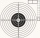The shooter shoots at the target, assuming that the individual shots are independent of each other and the probability of hitting each of them is 0.2. The shooter fires until he hits the target for the first time, then stop firing. (a) What is the most li
• Bouquet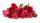Gardener tying bouquet of flowers for 8 and none was left. Then he found that he could tying bouquet of 6 flowers and also none was left. How many have gardener flowers (minimum and maximum) if they had between 50 and 100 flowers?

Do you have an interesting mathematical word problem that you can't solve it? Submit a math problem, and we can try to solve it.

We will send a solution to your e-mail address. Solved examples are also published here. Please enter the e-mail correctly and check whether you don't have a full mailbox.

Please do not submit problems from current active competitions such as Mathematical Olympiad, correspondence seminars etc...

Minimum - math word problems. Maximum - math word problems.